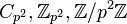# Cyclic group of prime-square order

## Definition

Let$p$ be a prime number. The cyclic group of order$p^2$, denoted$C_{p^2}, \mathbb{Z}_{p^2}, \mathbb{Z}/p^2\mathbb{Z}$, is defined as the cyclic group with$p^2$ elements.

This is the cyclic group corresponding to the partition$2$ of$2$.

## Particular cases

prime number$p$ corresponding cyclic group of prime-square order
2 cyclic group:Z4
3 cyclic group:Z9
5 cyclic group:Z25
7 cyclic group:Z49

## Arithmetic functions

Function Value Similar groups Explanation for function value
prime-base logarithm of order 2 groups with same prime-base logarithm of order
max-length of a group 2 max-length of a group equals prime-base logarithm of order for group of prime power order
chief length 2 chief length equals prime-base logarithm of order for group of prime power order
composition length 2 composition length equals prime-base logarithm of order for group of prime power order
prime-base logarithm of exponent 2 groups with same prime-base logarithm of order and prime-base logarithm of exponent | groups with same prime-base logarithm of exponent
Frattini length 2 groups with same prime-base logarithm of order and Frattini length | groups with same Frattini length Frattini length equals prime-base logarithm of exponent for abelian group of prime power order
minimum size of generating set 1 groups with same prime-base logarithm of order and minimum size of generating set | groups with same minimum size of generating set
subgroup rank of a group 1 groups with same prime-base logarithm of order and subgroup rank of a group | groups with same subgroup rank of a group same as minimum size of generating set since it is an abelian group of prime power order
rank of a p-group 1 groups with same prime-base logarithm of order and rank of a p-group | groups with same rank of a p-group same as minimum size of generating set since it is an abelian group of prime power order
normal rank of a p-group 1 groups with same prime-base logarithm of order and normal rank of a p-group | groups with same normal rank of a p-group same as minimum size of generating set since it is an abelian group of prime power order
characteristic rank of a p-group 1 groups with same prime-base logarithm of order and characteristic rank of a p-group | groups with same characteristic rank of a p-group same as minimum size of generating set since it is an abelian group of prime power order
nilpotency class 1 The group is a nontrivial abelian group
derived length 1 The group is a nontrivial abelian group
Fitting length 1 The group is a nontrivial abelian group

## GAP implementation

### Group ID

This finite group has order p^2 and has ID 1 among the group of order p^2 in GAP's SmallGroup library. It can thus be defined using GAP's SmallGroup function as:

SmallGroup(p^2,1)

For instance, we can use the following assignment in GAP to create the group and name it$G$:

gap> G := SmallGroup(p^2,1);

Conversely, to check whether a given group$G$ is in fact the group we want, we can use GAP's IdGroup function:

IdGroup(G) = [p^2,1]

or just do:

IdGroup(G)

to have GAP output the group ID, that we can then compare to what we want.